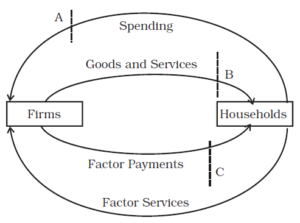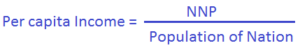# National Income Definition, Measurement, GDP, GNP, NDP

National Income of country is the money value of goods and services produced over the year. There are many sorts of national income definition but we will discuss some of the common national income definition like GDP, GNP etc.The measurement of national income of an economy can be done using three methods:

• Value Added or Product Method
• Expenditure Method
• Income Method

Let’s understand these three methods one by one.

### Value Added or Product Method

According to value added method of calculating national income, the national income is the aggregate money value of goods and services produced over the period of one year. The value of intermediate goods consumed in the process of production is subtracted from total production. Thus,

Value Added = Value of production – value of intermediate goods

The value added by firm is distributed among its four factors of production:

• Land (in the form of Rent)
• Labour (in the form of salary and wages)
• Capital (in the form of interest)
• Entrepreneurship (in the form of profit)

What are Intermediate Goods?

Intermediate Goods are the raw materials that a firm buys from another firm which are completely used up in the process of production.

Now let us understand the concept of value added with the help of an example, suppose there are two products viz milk and cheese produced in the economy. Let’s say money value of milk produced in the year is Rs 200 and money value of cheese produced over the course of year is Rs 300. As we know cheese is produced from milk, so there must be consumption of some milk in production of cheese thus milk acts as intermediate good for production of cheese. Let’s money value of milk used for production of cheese is Rs 100.

Now, the value added of economy = Rs 200 (milk produced) + Rs 300 (cheese produced) – Rs 100 (intermediate good milk used for cheese production) = Rs 400.

What is depreciation?

The depreciation is the reduction in value of asset due to its age, wear and tear, obsolescence happened to fixed asset while goods and services are being produced..

Suppose in our above example of milk and cheese. The cheese is produced using a machine whose cost of buying is Rs 100. Every machine has some age and due its wear and tear after some point of time the machine became obsolete.  Let’s say machine loses its 10% value every year i.e depreciation rate is 10%. Therefore cheese producing machine will loose 10% of its value very year

Depreciation = 10% of Rs 100 = Rs 10.

We must understand that depreciation is an accounting concept and it is not actual expense. It is charged keeping in mind that an asset needs to get replaced after its life is over.

Gross value added (GVA) is the total of value added and depreciation of fixed capital.

i.e.  GVA = value added + depreciation

In our above milk-cheese example, the gross value added = Rs 400 + Rs 10 = Rs 410.

Conversely, Net Value added is obtained by subtracting depreciation from Gross Value Added.

In our above milk-cheese example, the net value added = Rs 410 – Rs 10 = Rs 400.

### Expenditure Method

As per expenditure method of calculating national income, the national income is the sum total of all expenditure made on purchase of final goods and services produced during the year.  It includes:

• Personal Consumption expenditure by household (C) on buying goods and services
• Firms on acquiring capital goods (I) like machinery, land and equipment etc
• Expenditure by government on final goods and services (G) to fulfill social welfare
• Expenditure on Net export (difference between expenditure on export (X) and import (M))

National Income = C + I + G + (X – M)

### Income Method

According to Income method of calculating national income, the national income is the income received by factors of production viz wages (W), rent (R), interest (I) and profit (P).

National Income = W + R + I + P

## Other National Income Definition

Let’s take a look at some other National income definition.

### Gross Domestic Product (GDP)

Gross Domestic Product is the value of all the final goods and services produced within the boundary of a nation during one year period. The GDP is used to express growth rate of an economy.  For example, if last year GDP of country is Rs 100 and this year, it has increased to Rs 110, then the growth rate of GDP is 10%. It is a quantitative concept and does not tell us anything about quality of goods and services produced. GDP is one of the widely used national income definition.

Nominal GDP
Nominal GDP is the value of goods and services produced during a given year valued at the prices that prevailed in that same year

Real GDP
Real GDP is the value of final goods and services produced in a given year when valued at constant prices.

### Net Domestic Product (NDP)

NDP is the GDP calculated after adjusting the value of depreciation.

NDP = GDP – Depreciation

Thus NDP of an economy must always be lower than GDP.

### Gross National Product (GNP)

Gross Nation Product (GNP) is GDP plus ‘Income from Abroad’. The ‘Income from Abroad’ has three sources:

• Trade Balance: The net outcome of total export and import at the year is added. This can be positive or negative depending upon the volume of export/import.
• Interest on loans: The net outcome of interest payment i.e. inflow due to money lent and outflow due to money borrowed. In India’s case net of interest payment is negative since India has more borrowings than lending.
• Private Remittances: The net outcome of inflows by private transfer from Indian nationals working in other countries and outflows by foreign nationals working in India. India is one of the highest receivers of private remittances.

The sum of these three components constitutes ‘Income from Abroad’. In India’s case ‘Income from Abroad’ has always been negative.

GNP = GDP + ‘Income from Abroad’

Therefore GNP in India is always lower than its GDP.

### Net National Product

NNP is the GNP calculated after adjusting the value of depreciation. This is also called National Income (NI) of an economy.

NNP = GNP – Depreciation
Or
NNP = GDP + Income from Abroad – Depreciation

Thus NNP of an economy must always be lower than GNP.

### Per capita Income

Per capita income of nation is calculated by dividing NNP by population of nation.Here are some useful articles for you to read next:

Economic Growth and Development
Types of Unemployment
Distribution Network Models

Click to go to RBI Grade B Preparation Page